# Calculate the torque needed to move a lead screw

Summary:: Calculate the torque needed to move a load with a lead screw. Torque is needed in order to find the right DC motor and coupling.

Hello,

I have a design with using a lead screw to move a load (see sketch below). The load will operate in both directions, in order to select the right DC motor and coupling (flexible coupling) I want to calculate how many torque is needed to move the load (for both directions. I found out the following and hope I am on the right way:
1. Material nut = CuSn7ZnPb (Bronze)
2. Material spindle = C45 (Steel)
3. Friction for Steel/Bronze according Shigley = 0.2
4. Load (F) = 1000 N = 1000/2 = 500 N
5. Spindle and nut are Tr12 x 2
From my calculation I found a needed torque of 0.73 Nm. Is this correct? Besides, is this torque constantly needed of is this the peak torque ?

Calculation: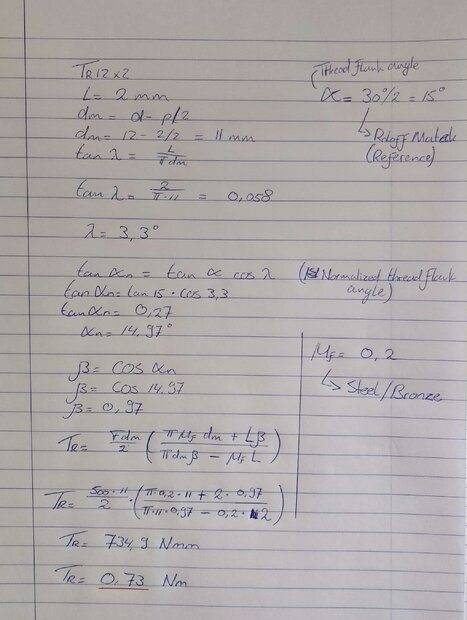Sketch: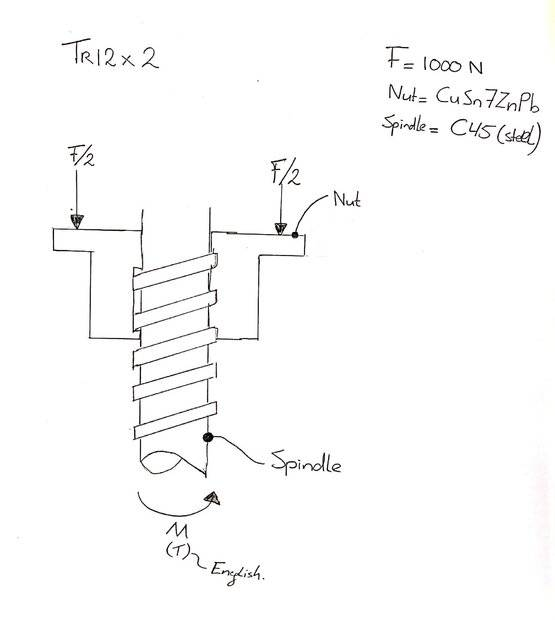#### Attachments

•Delta2

According to Shigley’s Mechanical Engineering Design the formula is: $$T_{R}=\frac{F d_{m}}{2} \left( \frac{l+ \pi f d_{m} sec \alpha}{\pi d_{m}- fl sec \alpha} \right)$$
where: ##F## - axial force, ##d_{m}## - mean diameter, ##l## - lead, ##f## - friction coefficient, ##\alpha## - half of the thread angle.

jrmichler
Mentor
The quick and easy way to cross check your torque calculation is to search torque tension chart. Look for the closest standard thread, then scale the results to your load. A 12 X 1.75 mm bolt with 500 N load came to slightly more than 1 N-m torque. That is for a V-thread bolt, so your calculation of 0.73 N-m for a square thread looks good.

If you are turning the screw, then you need to add the friction between the screw and the load. If turning the nut, there is bearing friction for the nut.

Your friction value of 0.2 is for smooth steel against smooth bronze, well lubricated, and while moving. If the screw thread is machined (not ground), it is not smooth. Static friction is typically higher than dynamic friction, especially if it sits without moving for long times (hours or days). A safety factor is a good idea. I suggest a friction value of 0.5 to account for these factors, which would increase your design torque to 1.8 N-m.

••jim mcnamara, Mech_LS24 and Twigg

@FEAnalyst, could you explain what the difference is between the two formula's? The definition of sec isn't yet known to me. I see on the internet it is as follows: sec x = 1/cos x, so why not use the formula with the cosinus and sinus included? And what can you say about the torque which is calculated? Is this torque constantly needed to move the load?

@jrmichler, thank you for the clear explanation. I search at the web and find a torque tension chart here: Torque Tension Chart. How did you find that torque of 1 Nm for a 500 N load? I do understand the scaling you did, but which thread class is realistic? Between the 8.8 and 12.9 the difference is ± 30 ft-lbs. K is the torque coefficient, which is the same as the friction?

Your friction value of 0.2 is for smooth steel against smooth bronze, well lubricated, and while moving. If the screw thread is machined (not ground), it is not smooth. Static friction is typically higher than dynamic friction, especially if it sits without moving for long times (hours or days). A safety factor is a good idea. I suggest a friction value of 0.5 to account for these factors, which would increase your design torque to 1.8 N-m.
Thank you for this! Gives me a nice practical view :)

BTW, using this formula below gives the value of 0.74 Nm (instead of the 0.73 Nm).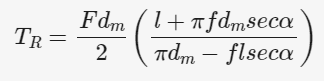@FEAnalyst, could you explain what the difference is between the two formula's? The definition of sec isn't yet known to me. I see on the internet it is as follows: sec x = 1/cos x, so why not use the formula with the cosinus and sinus included? And what can you say about the torque which is calculated? Is this torque constantly needed to move the load?
In this formula sec stands for secant which is just another trigonometric function defined here: https://en.wikipedia.org/wiki/Trigonometric_functions

It's like with every force that has to overcome friction. Imagine a box being pushed on the floor. Is the force required only at the beginning or all the time ? However, you should also consider a difference between static and kinetic friction coefficient. Let me cite this website: https://www.engineersedge.com/mechanics_machines/power_screws_design_13982.htm

The starting torque is obtained by substituting the static coefficients of friction into the above equations. Since the sliding coefficient of friction is roughly 25 percent less than the static coefficient, the running torque is somewhat less than the starting torque.

•Mech_LS24
Load (F) = 1000 N = 1000/2 = 500 N

You seem to have divided your required force in half for your calculation. Do you have two of these power screws in parallel?

Thanks @FEAnalyst.

I can imagine now the torque which is calculated can be considered as the highest torque needed in this application. As the quote says: "Since the sliding coefficient of friction is roughly 25 percent less than the static coefficient, the running torque is somewhat less than the starting torque."

What I was also wondering, should I take into account collar bearing calculation? As shown in the sketch in the first post, I don't think it's the case here. Or do I miss something here? Torque collar bearing formula: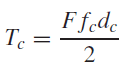You seem to have divided your required force in half for your calculation. Do you have two of these power screws in parallel?
Because I have a force of 1000 N loaded at the spindle, this results in the F/2 as shown in the image below: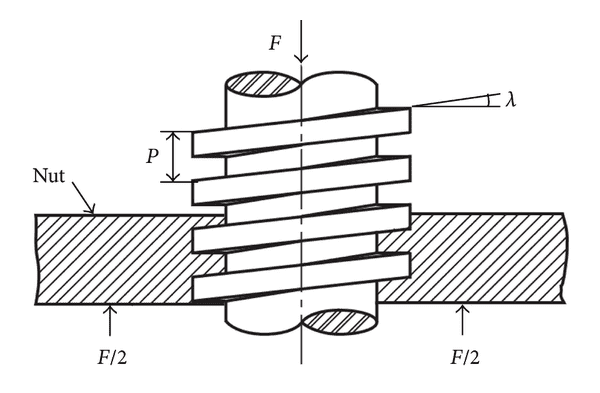this results in the F/2
this results in 2 x F/2 = F.

You're still effecting a total force of F, which has to be accounted for. If you're only considering the torque required for one side of the nut, you'd still have to multiply by 2 to account for the other side as well.

The derivation is done by unrolling the screw, which would wrap both (F/2) into the total F. Notice that the base of the triangle is the circumference of the screw. Both sides are included in F.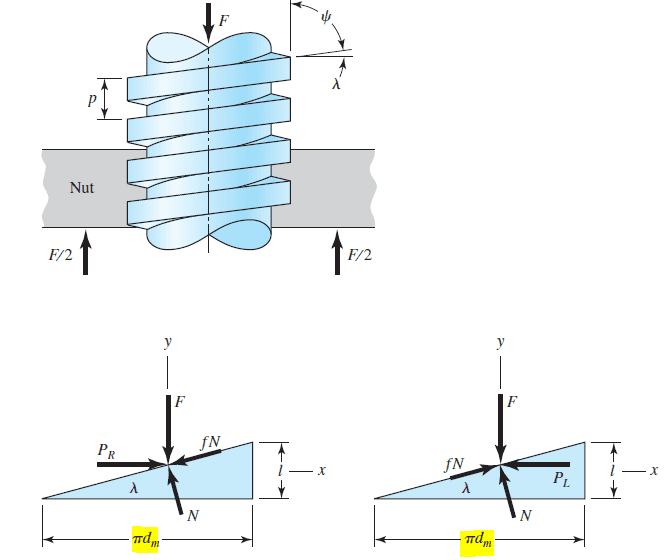•Mech_LS24
The derivation is done by unrolling the screw, which would wrap both (F/2) into the total F. Notice that the base of the triangle is the circumference of the screw. Both sides are included in F.

Thank you Onatirec. Should I also take the collar friction into account? It would have a significant affect on the total torque.

It's not clear from your diagram that you have a collar on the screw. If you have one, you should account for the friction; if not, then no.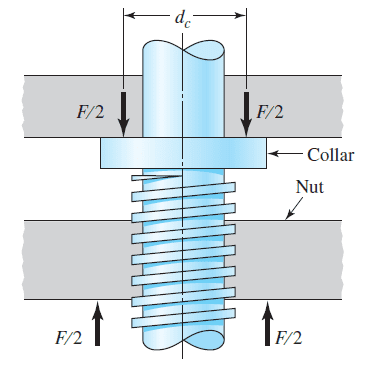It's not clear from your diagram that you have a collar on the screw.
This (below) is what I have. The flange where the nut is mounted on, should been seen as the collar I assume?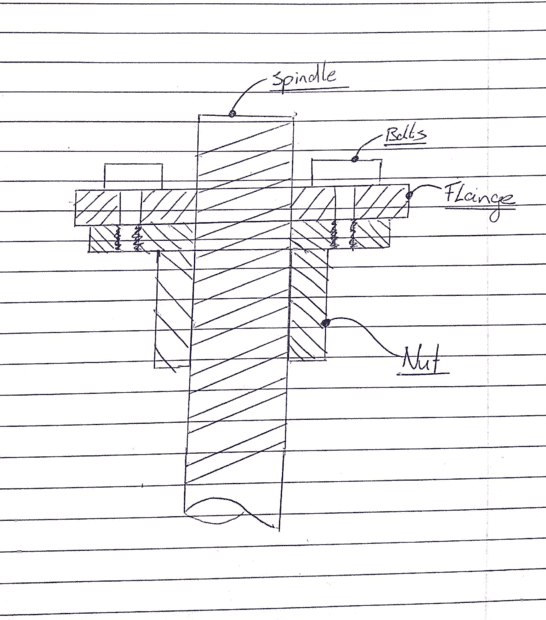You can see in my post #12 above that the collar is attached to the screw and spins against the gray surface above it. The additional friction between those two surfaces is what the collar torque (Tc) has to overcome.

In your diagram the flange is bolted to the nut. So there doesn't appear to be any additional frictional surfaces protruding from the screw (in addition to the threads).

At the end of the day though, it's going to be better to have a slightly oversized motor than an undersized one.

•Mech_LS24
You can see in my post #12 above that the collar is attached to the screw and spins against the gray surface above it. The additional friction between those two surfaces is what the collar torque (Tc) has to overcome.
Thanks, this means that the torque Tc only should been considered when a collar turns against a surface? Any 'fixed' joint/connection doesn't apply the Tc torque. Is there an practical example for this? I can imagine it has something to do with bearings combined with the nut or so.

On the internet I have seen "anti backlash nuts" Anti backlash nut example (see image), I searched on the forum but couldn't find any related topics. But it has made me interested how this should be considered as there is (in my opinion) another force namely, the Force occurred due to the spring (Fs). Does it makes the sketch with the forces as drawn below? Should this spring force be added to the axial load force? So this will increase the total axial load force?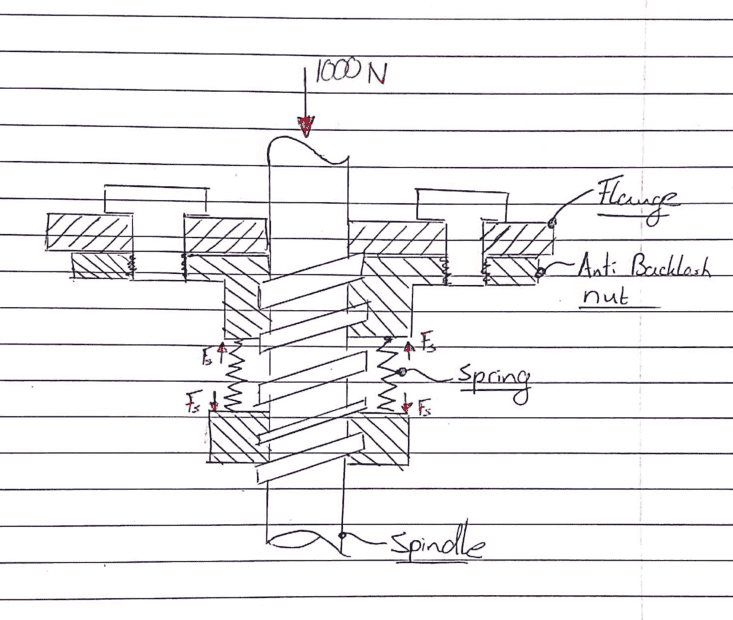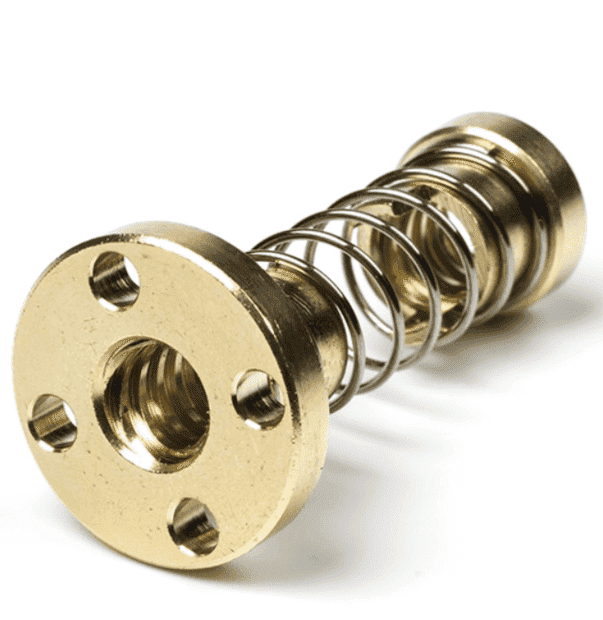Is there anyone who can help with this thread? Thanks

jrmichler
Mentor
Some points:

1) A solid part with a load, such as a screw or nut, with a force on it has one force F. It can be shown as two forces, where each force is F/2, in order to make it clear to which part the force is applied. The total force on the part is then 2 * F/2 = F.

2) Assume a screw with a force F. In the homework world, that force is applied against a nut, and the assigned problem is to calculate the torque given a friction coefficient. The screw is rotating in space, and the force is applied by magic. There is one calculation of friction.

In the real world, something is applying that force to the screw. That something can be the end of the screw pushing against something else, a collar, or it can be a bearing. That something has a friction that can be calculated, and adds to the torque required to rotate the screw. There are at least two calculations of friction, with the results summed.

3) The diagram in Post #12 clearly shows the screw load being applied by a collar on the screw. The forces F/2 should be one force F on each part. Splitting the force F into two forces F/2 is confusing. Although it is difficult to properly show a single force F on each part in a simple diagram.

4) The diagram in Post #13 is confusing. It does not show what part is carrying what load.

5) I suggest that the OP clarify whether this is a simple homework problem, a simple homework problem that lead to questions about collars, and if they now understand screw threads and friction.

6) Post #15 should be in a new thread. It's a separate topic from the earlier posts.

Hi @jrmichler! thank you for your reply. It makes sense to me that the force is F/2 is more or less a representation of the total Force F now.

I agree with you that the last post probably should be in the Mechanical Engineering forum. Can I move it myself to this forum? This topic starts more or less with a homework problem but the more I know now, the more interesting 'other' things are. I would like to figure out how it all works :).